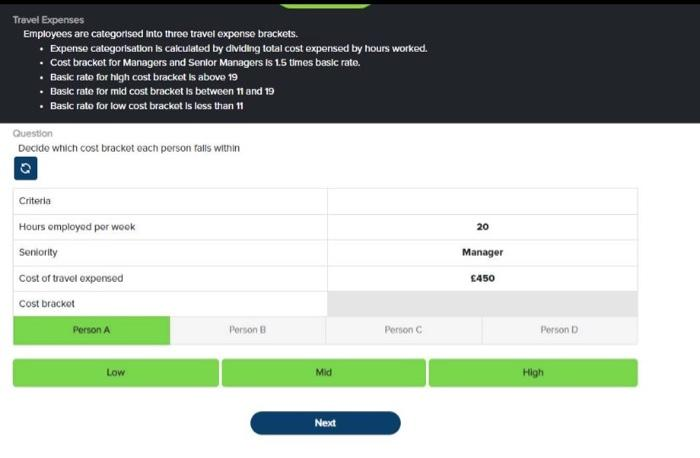# Question Trevel Expenses Employees are categorised into three travel expense brackets. - Expense categorisatlon is calculated by dividing total cost expensed by hours worked. - Cost bracket for Managers and Senlor Managers is $$1.5$$ times basic rate. - Basic rate for high cost bracket is above 19 - Basic rate for mid cost bracket is between $$\mathbf{1 1}$$ and $$\boldsymbol{1 9}$$ - Basic rato for low cost brackot is loss than 11Transcribed Image Text: Trevel Expenses Employees are categorised into three travel expense brackets. - Expense categorisatlon is calculated by dividing total cost expensed by hours worked. - Cost bracket for Managers and Senlor Managers is $$1.5$$ times basic rate. - Basic rate for high cost bracket is above 19 - Basic rate for mid cost bracket is between $$\mathbf{1 1}$$ and $$\boldsymbol{1 9}$$ - Basic rato for low cost brackot is loss than 11
Transcribed Image Text: Trevel Expenses Employees are categorised into three travel expense brackets. - Expense categorisatlon is calculated by dividing total cost expensed by hours worked. - Cost bracket for Managers and Senlor Managers is $$1.5$$ times basic rate. - Basic rate for high cost bracket is above 19 - Basic rate for mid cost bracket is between $$\mathbf{1 1}$$ and $$\boldsymbol{1 9}$$ - Basic rato for low cost brackot is loss than 11&#12304;General guidance&#12305;The answer provided below has been developed in a clear step by step manner.Step1/2We have the data for Person A i.e.\begin{align*} \mathrm{\text{Hours employed per week}} &= \mathrm{{20}} \\[3pt]\mathrm{\text{Seniority}} &= \mathrm{\text{Manager}} \\[3pt]\mathrm{\text{Cost of travel expensed}} &= \mathrm{\{450}} \end{align*}Now it is given that Expense categorisation is calculated by dividing total cost expensed by hours worked.So\begin{align*} \mathrm{\text{Expense categorisation}} &= \mathrm{\frac{{450}}{{20}}} \\[3pt] &= \mathrm{{22.5}} \end{align*}Explanation:Please refe ... See the full answer